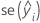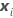# Methods and formulas for marginal fits and residuals in Fit Mixed Effects Model

Select the method or formula of your choice.

## Marginal fitted value

The predicted y or; the mean response value for the given the values of the fixed factors, and covariates using the marginal fitted equation. The marginal equation does not include coefficients for any random factors.

For further details on the notation, go to the Methods section and the Test of Fixed Effects section.

## Standard error of the marginal fitted value (SE Fit)

The standard error of the marginal fitted values in the mixed model depend on the test method for the fixed effects. For both methods, the standard errors are the square roots of the diagonal elements of the variance matrix of the fits.

where

where

## Confidence intervals for marginal means

The confidence interval for themarginal mean response is calculated using the following expression:is the marginal fit.is the standard error of the fit.

The degrees of freedom use this formula for the marginal case:

where

### Notation

TermDescriptiontherow of the X matrix

## Marginal residuals

Themarginal residual is calculated by the following expression:

### Notation

TermDescription
yitheresponse valuethemarginal fitted value

## Standardized residuals

Standardized residuals are also called "internally Studentized residuals."

where the standard deviation of the residual is the appropriate diagonal square root of the residual variance matrix:

where

### Notation

TermDescription
eithe ith residual
Std(ei)the standard deviation of the ith residual

## Leverages (Hi) for marginal data

The leverage matrix has the following form:

The Hi column displays the diagonal elements of H.

By using this site you agree to the use of cookies for analytics and personalized content.  Read our policy